The VARMAX Procedure

Parameter Estimation and Testing on Restrictions

In the previous example, the VARX(1,0) model is written aswith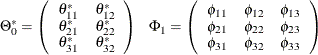In Figure 35.20 of the preceding section, you can see several insignificant parameters. For example, the coefficients XL0_1_2, AR1_1_2, and AR1_3_2 are insignificant.

The following statements restrict the coefficients of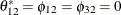for the VARX(1,0) model.

/*--- Models with Restrictions and Tests ---*/

proc varmax data=grunfeld;
model y1-y3 = x1 x2 / p=1 print=(estimates);
restrict XL(0,1,2)=0, AR(1,1,2)=0, AR(1,3,2)=0;
run;


The output in Figure 35.21 shows that three parameters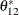,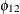, and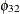are replaced by the restricted values, zeros. In the schematic representation of parameter estimates, the three restricted parameters,, andare replaced by.

Figure 35.21: Parameter Estimation with Restrictions

The VARMAX Procedure

XLag
Lag Variable x1 x2
0 y1 1.67592 0.00000
y2 -6.30880 2.65308
y3 -0.03576 -0.00919

AR
Lag Variable y1 y2 y3
1 y1 0.27671 0.00000 0.01747
y2 -2.16968 0.10945 -0.93053
y3 0.96398 0.00000 0.93412

Schematic Representation
Variable/Lag C XL0 AR1
y1 . +* ...
y2 + .+ ..-
y3 - .. +*+

The output in Figure 35.22 shows the estimates of the Lagrangian parameters and their significance. Based on the p-values associated with the Lagrangian parameters, you cannot reject the null hypotheses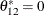,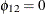, and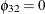with the 0.05 significance level.

Figure 35.22: RESTRICT Statement Results

Testing of the Restricted Parameters
Parameter Estimate Standard
Error
t Value Pr > |t| Equation
Restrict0 1.74969 21.44026 0.08 0.9389 XL0_1_2 = 0
Restrict1 30.36254 70.74347 0.43 0.6899 AR1_1_2 = 0
Restrict2 55.42191 164.03075 0.34 0.7524 AR1_3_2 = 0

The TEST statement in the following example tests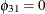andfor the VARX(1,0) model:

proc varmax data=grunfeld;
model y1-y3 = x1 x2 / p=1;
test AR(1,3,1)=0;
test XL(0,1,2)=0, AR(1,1,2)=0, AR(1,3,2)=0;
run;


The output in Figure 35.23 shows that the first column in the output is the index corresponding to each TEST statement. You can reject the hypothesis testat the 0.05 significance level, but you cannot reject the joint hypothesis testat the 0.05 significance level.

Figure 35.23: TEST Statement Results

The VARMAX Procedure

Testing of the Parameters
Test DF Chi-Square Pr > ChiSq
1 1 150.31 <.0001
2 3 0.34 0.9522